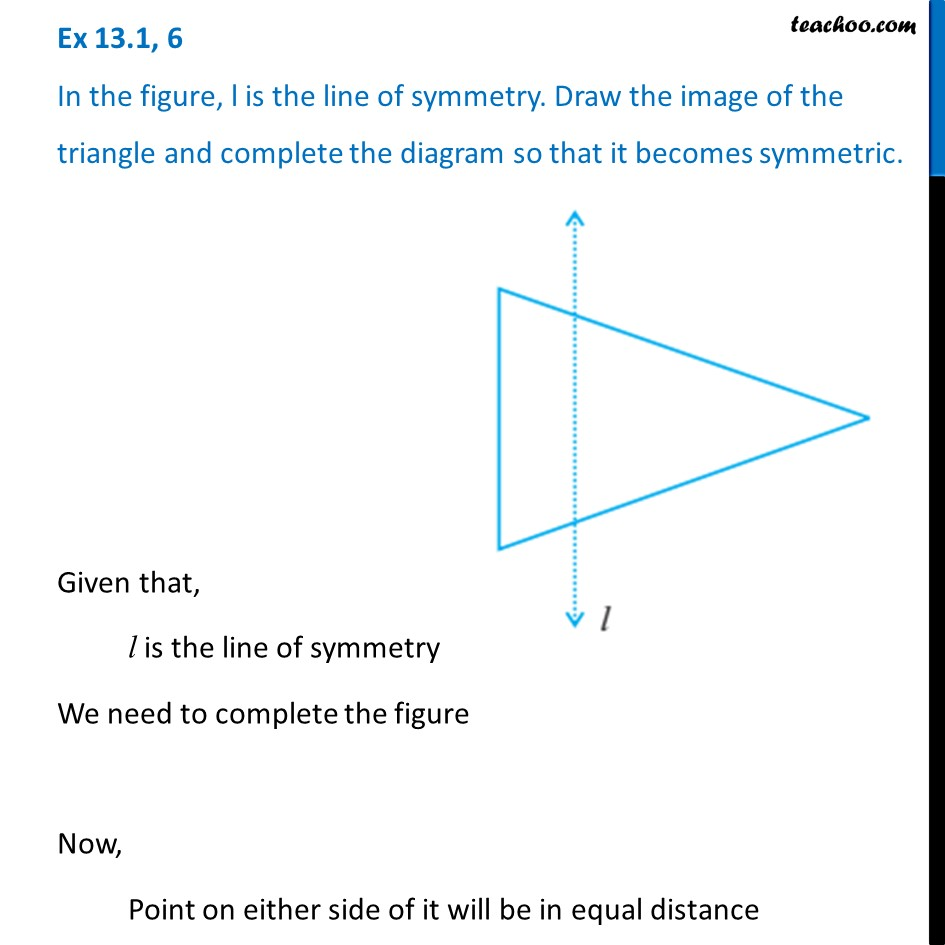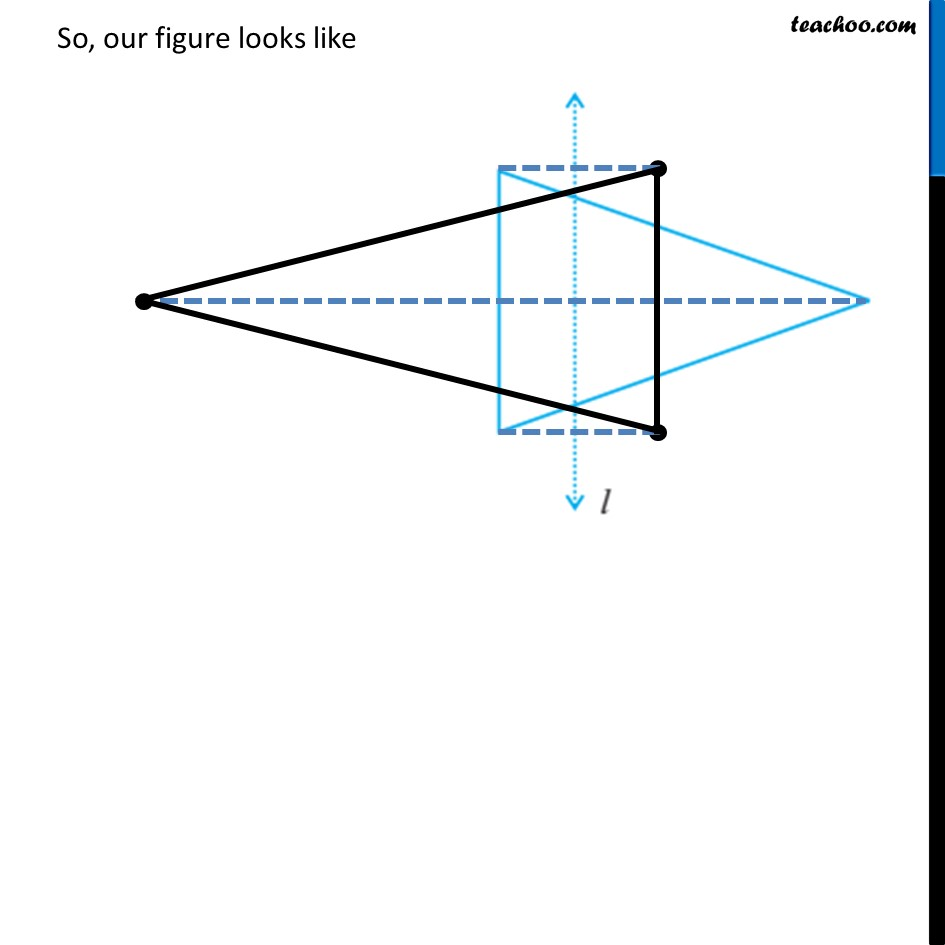Symmetry Definition

Symmetry
Serial order wiseLearn in your speed, with individual attention - Teachoo Maths 1-on-1 Class

### Transcript

Question 6 In the figure, l is the line of symmetry. Draw the image of the triangle and complete the diagram so that it becomes symmetric.Given that, l is the line of symmetry We need to complete the figure Now, Point on either side of it will be in equal distance So, our figure looks like